# 6.4 Combining Sources of Information

Throughout this chapter, we have seen perceptual processes that combine information from multiple sources. These could be cues from the same sense, as in the numerous monocular cues used to judge depth. Perception may also combine information from two or more senses. For example, people typically combine both visual and auditory cues when speaking face to face. Information from both sources makes it easier to understand someone, especially if there is significant background noise. We have also seen that information is integrated over time, as in the case of saccades being employed to fixate on several object features. Finally, our memories and general expectations about the behavior of the surrounding world bias our conclusions. Thus, information is integrated from prior expectations and the reception of many cues, which may come from different senses at different times.

Statistical decision theory provides a useful and straightforward mathematical model for making choices that incorporate prior biases and sources of relevant, observed data. It has been applied in many fields, including economics, psychology, signal processing, and computer science. One key component is Bayes' rule, which specifies how the prior beliefs should be updated in light of new observations, to obtain posterior beliefs. More formally, the beliefs'' are referred as probabilities. If the probability takes into account information from previous information, it is called a conditional probability. There is no room to properly introduce probability theory here; only the basic ideas are given to provide some intuition without the rigor. For further study, find an online course or classic textbook (for example, ).

Let(6.1)

be a set of hypotheses (or interpretations). Similarly, let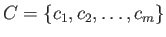(6.2)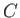be a set of possible outputs of a cue detector. For example, the cue detector might output the eye color of a face that is currently visible. In this caseis the set of possible colors: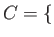BROWN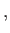BLUEGREENHAZEL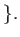(6.3)

Modeling a face recognizer,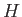would correspond to the set of people familiar to the person.

We want to calculate probability values for each of the hypotheses in. Each probability value must lie between 0 to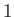, and the sum of the probability values for every hypothesis inmust sum to one. Before any cues, we start with an assignment of values called the prior distribution, which is written as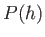. The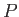'' denotes that it is a probability function or assignment;means that an assignment has been applied to every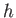in. The assignment must be made so that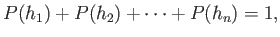(6.4)

and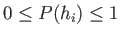for each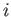fromto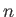.

The prior probabilities are generally distributed across the hypotheses in a diffuse way; an example is shown in Figure 6.25(a). The likelihood of any hypothesis being true before any cues is proportional to its frequency of occurring naturally, based on evolution and the lifetime of experiences of the person. For example, if you open your eyes at a random time in your life, what is the likelihood of seeing a human being versus a wild boar?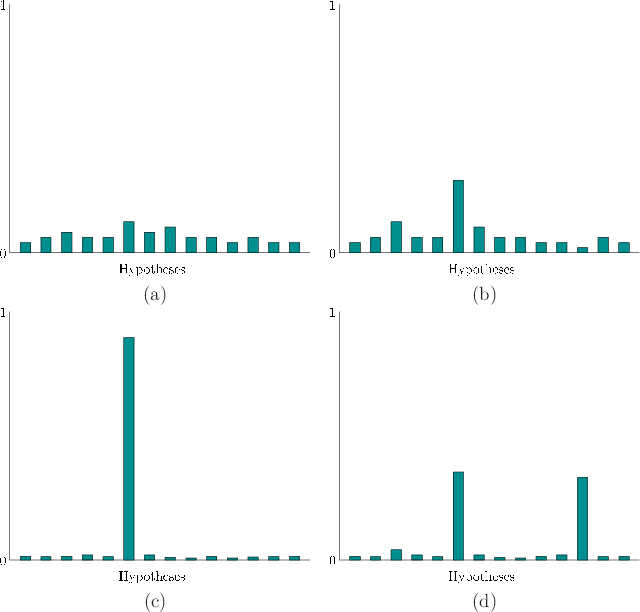Under normal circumstances (not VR!), we expect that the probability for the correct interpretation will rise as cues arrive. The probability of the correct hypothesis should pull upward toward, effectively stealing probability mass from the other hypotheses, which pushes their values toward 0; see Figure 6.25(b). A strong'' cue should lift the correct hypothesis upward more quickly than a weak'' cue. If a single hypothesis has a probability value close to, then the distribution is considered peaked, which implies high confidence; see Figure 6.25(c). In the other direction, inconsistent or incorrect cues have the effect of diffusing the probability across two or more hypotheses. Thus, the probability of the correct hypothesis may be lowered as other hypotheses are considered plausible and receive higher values. It may also be possible that two alternative hypotheses remain strong due to ambiguity that cannot be solved from the given cues; see Figure 6.25(d).

To take into account information from a cue, a conditional distribution is defined, which is written as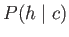. This is spoken as the probability ofgiven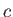.'' This corresponds to a probability assignment for all possible combinations of hypotheses and cues. For example, it would include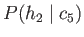, if there are at least two hypotheses and five cues. Continuing our face recognizer, this would look like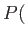BARACK OBAMA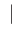BROWN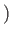, which should be larger thanBARACK OBAMABLUE(he has brown eyes).

We now arrive at the fundamental problem, which is to calculateafter the cue arrives. This is accomplished by Bayes' rule: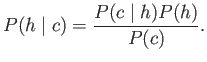(6.5)

The denominator can be expressed as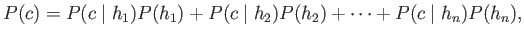(6.6)

or it can be ignored it as a normalization constant, at which point only relative likelihoods are calculated instead of proper probabilities.

The only thing accomplished by Bayes' rule was to expressin terms of the prior distributionand a new conditional distribution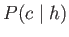. The new conditional distribution is easy to work with in terms of modeling. It characterizes the likelihood that each specific cue will appear given that the hypothesis is true.

What if information arrives from a second cue detector? In this case, (6.5) is applied again, butis now considered the prior distribution with respect to the new information. Let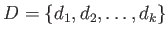represent the possible outputs of the new cue detector. Bayes' rule becomes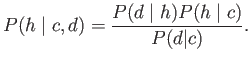(6.7)

Above,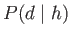makes what is called a conditional independence assumption: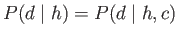. This is simpler from a modeling perspective. More generally, all four conditional parts of (6.7) should containbecause it is given before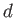arrives. As information from even more cues becomes available, Bayes' rule is applied again as many times as needed. One difficulty that occurs in practice and modeled here is cognitive bias, which corresponds to numerous ways in which humans make irrational judgments in spite of the probabilistic implications of the data.

Subsections
Steven M LaValle 2019-03-14